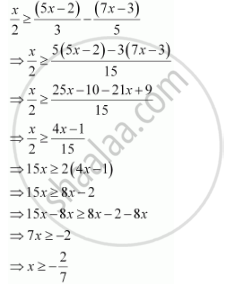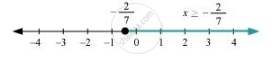# Solve the Given Inequality and Show the Graph of the Solution on Number Line X/2 >= (5x -2)/3 - (7x - 3)/5 - Mathematics

Solve the given inequality and show the graph of the solution on number line x/2 >= (5x -2)/3 - (7x - 3)/5

#### SolutionThe graphical representation of the solutions of the given inequality is as follows.Concept: Algebraic Solutions of Linear Inequalities in One Variable and Their Graphical Representation
Is there an error in this question or solution?
Chapter 6: Linear Inequalities - Exercise 6.1 [Page 122]

#### APPEARS IN

NCERT Class 11 Mathematics
Chapter 6 Linear Inequalities
Exercise 6.1 | Q 20 | Page 122
Share